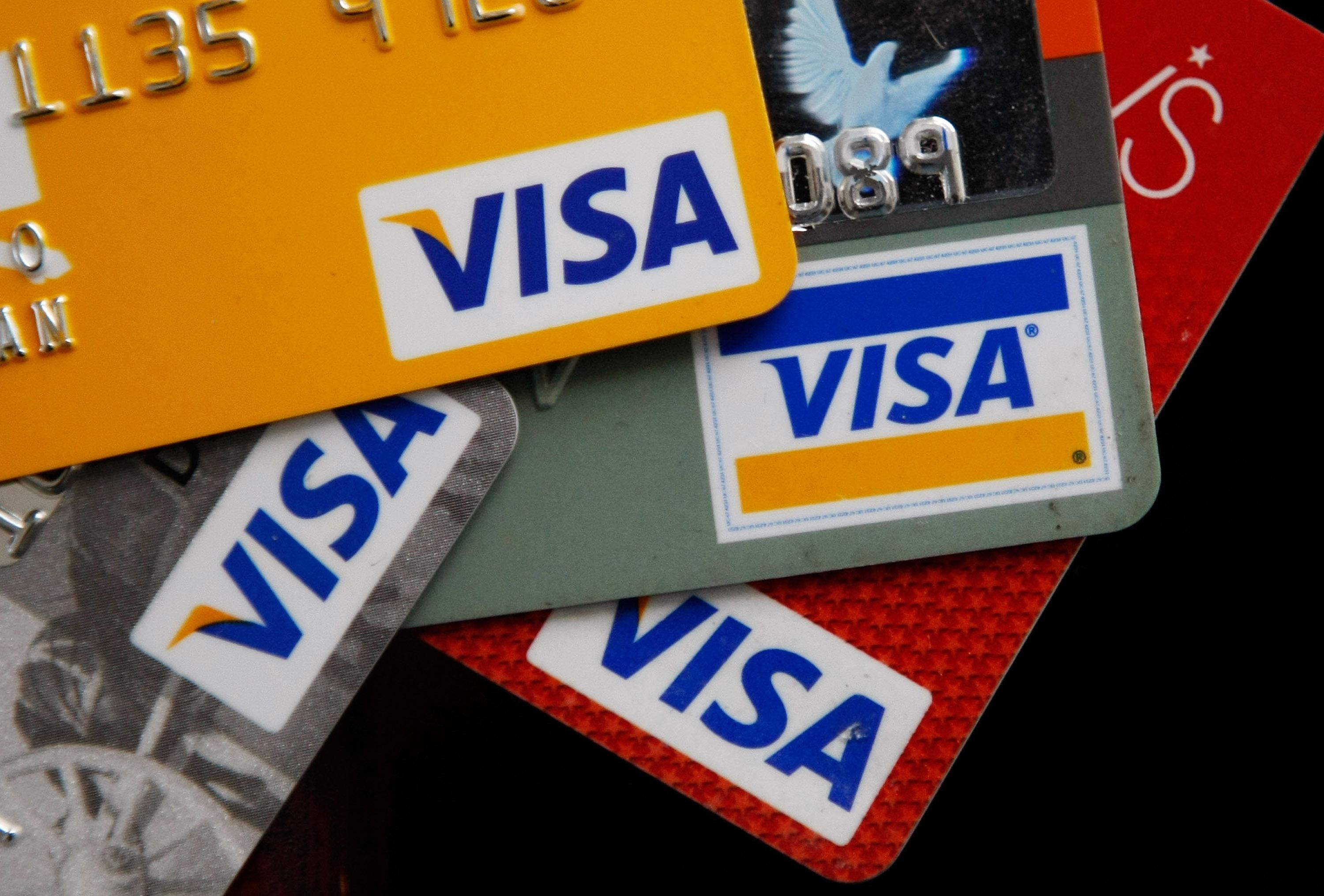Science

# Prime Numbers Hide Your Secrets

## What happens when you enter your credit card number online?How exactly are credit card purchases kept secure? Photo Illustration by Justin Sullivan/Getty Images

Prime numbers are all the rage these days. I can tell something’s up when random people start asking me about the randomness of primes—without even knowing that I’m a mathematician! In the past couple of weeks we’ve heard about a beautiful result on the gaps between primes and about cicadas’ prime-numbered life cycles. Our current love affair with primes notwithstanding, many people have wondered whether this is all just abstract theoretical stuff or whether prime numbers have real-world applications.

In fact, they have applications to something as ubiquitous and mundane as making a purchase online. Every time you enter your credit card number on the Internet, prime numbers spring into action. Before your card number is sent over the wires, it must be encrypted for security, and once it’s received by the merchant, it must be decrypted. One of the most common encryption schemes, the RSA algorithm, is based on prime numbers. It uses a “public key,” information that is publicly available, and a “private key,” something that only the decoding party (merchant) has. Roughly speaking, the public key consists of a large number that is the product of two primes, and the private key consists of those two primes themselves. It’s very difficult to factor a given large number into primes. For example, it took researchers two years recently to factor a 232-digit number, even with hundreds of parallel computers. That’s why the RSA algorithm is so effective.

Prime numbers are whole numbers greater than 1 that are not divisible by any whole number other than 1 and itself. The first few are 2, 3, 5, 7, 11, 13 … To explain how the RSA algorithm works, I need to tell you first about something called Fermat’s little theorem. It was discovered by Pierre Fermat, the same French mathematician who came up with the famous Fermat’s last theorem. Fermat had a penchant for being cryptic; in the case of his last theorem, he left a note on the margin of a book stating his theorem and adding: “I have discovered a truly marvelous proof of this, which this margin is too narrow to contain.” Call it the 17th-century version of a Twitter proof. Many professional mathematicians and amateurs tried to reproduce Fermat’s purported proof, and it took more than 350 years to come up with a real one.

To understand what Fermat’s little theorem means, we need to learn how to do arithmetic “modulo N.” This is something, in fact, we do all the time when adding angles. If you rotate an object by 180 degrees, and then by another 270 degrees, the net result will be rotation by 90 degrees. That’s because 180 + 270 = 450, and then we subtract 360 from it, because rotation by 360 degrees means no net rotation at all. This is what mathematicians call addition “modulo 360.” Likewise, we can do addition modulo any whole number N instead of 360. (Another familiar example is adding hours, where N = 12.) And we can also do multiplication modulo N.

Now suppose that N is a prime number. Then we have the following remarkable fact: Raising any number to the Nth power modulo N, we get back that same number. For example, if N = 5, then the 5th power of 1 is 1 and the 5th power of 2 is 32, which is equal to 2 modulo 5 because after you subtract the closest multiple of 5 (in this case, you subtract 30, or 5 × 6), you are left with 2 (because 32 = 5 × 6 + 2). Likewise, the 5th power of 3 is equal to 3 modulo 5, and so on. This is Fermat’s little theorem. Fermat first divulged it in a letter to a friend. “I would send you a proof of it,” he wrote, “but I am afraid it’s too long.” He was such a tease, this Fermat. Unlike the proof of his last theorem, however, the proof of the little one is surprisingly simple—it could even fit in the margin of a book. I would write it here, but my editor tells me that my article is already too long. No worries though, you can read the proof in this excerpt from my book Love and Math.

This is neat, but what does it have to with Internet security? We need to devise a two-step procedure: First encrypt a credit card number and then decrypt it, so that if we follow both steps we get back the original number. The good news from Fermat’s little theorem is that raising a card number to a prime power modulo that prime is a procedure that gives us back the original number. The bad news is that because a prime is not divisible by anything, there is no way to break this procedure into two steps. However, Ron Rivest, Adi Shamir, and Leonard Adleman, after whom the RSA algorithm is named, were not discouraged. They took Fermat’s idea one step further. They asked: What if we take N which is the product of two primes—call them p and q. Then we have the following analogue of Fermat’s little theorem: Raising any number to the power (p – 1)(q – 1) + 1 will give us back the same number modulo N. For example, N = 15 is the product of p = 3 and q = 5. Then (p – 1)(q – 1) + 1 = (3 – 1)(5 – 1) + 1 = 9. If you raise any number to the 9th power, you get back the same number modulo 15. It looks like a miracle, but in fact the proof is no more complicated than that of Fermat’s little theorem.